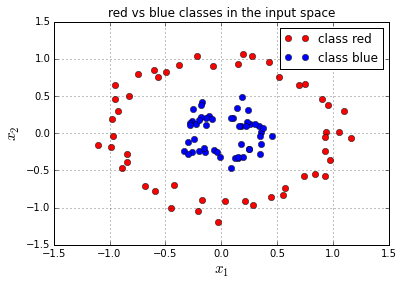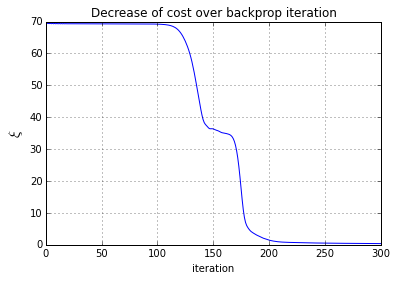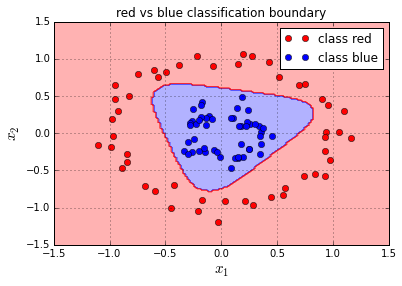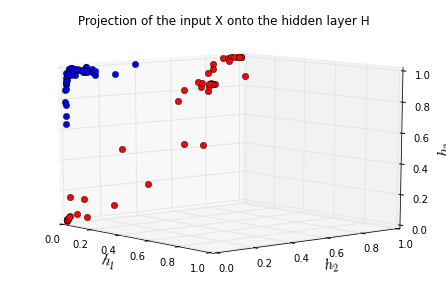# Good Articles to learn how to implement a neural network 2

Tags: , ,

## Vectorization

This part will cover:

The previous tutorial described a very simple neural network with only one input, one hidden neuron and one output. This tutorial will describe a neural network that takes 2-dimensional input samples, projects them onto a 3-dimensional hidden layer, and classifies them with a 2-dimensional softmax output classfier, this softmax function is explained in intermezzo 2 . While we didn’t add the bias parameters to the previous 2 models, we will add them to this model. We will work out the vector computations needed to optimize and run this neural network in this tutorial. The network of this model is shown in the following figure:The notebook starts out with importing the libraries we need:

In :

## Define the dataset

In this example the target classes t

corresponding to the inputs x will be generated from 2 class distributions: red (t=0) and blue (t=1

). Where the red class is a circular distribution that surrounds the distribution of the blue class. The dataset is generated by the scikit-learn make_circles method.

This results in a 2D dataset that is not linearly separable. The model from part 2 won’t be able to classify both classes correctly since it can learn only linear separators. By adding a hidden layer, the model will be able to train a non-linear separator.

The N

input samples with 2 variables each are given as a N×2 matrix X

:

X=⎡⎣⎢⎢⎢x11xN1x12xN2⎤⎦⎥⎥⎥

Where xij

is the value of the j-th variable of the i

-th input sample.

Since the softmax output function is used the targets for each input sample are given as a N×2

matrix T

:

T=⎡⎣⎢⎢⎢t11tN1t12tN2⎤⎦⎥⎥⎥

Where tij=1

if and only if the i-th input sample belongs to class j

. So blue points are labelled T = [0 1] and red points are labelled T = [1 0].

In :

In :## Vectorization of backpropagation

### 1. Vectorization of the forward step

#### Compute activations of hidden layer

The 2-dimensional inputs X

are projected onto the 3 dimensions of the hidden layer H by the weight matrix Wh (whij is the weight of the connection between input variable i and hidden neuron activation j) and bias vector bh

:

Wh=[wh11wh21wh12wh22wh13wh23]bh=[bh1bh2bh3]

following computation:

H=σ(XWh+bh)=11+e(XWh+bh)=⎡⎣⎢⎢⎢h11hN1h12hN2h13hN3⎤⎦⎥⎥⎥

With σ

the logistic function, and with H resulting in a N×3

matrix.

This computation flow is illustrated by the figure below. Each input xij

is multiplied with the weight parameters from the corresponding column whj1,whj2,whj3. The multiplication results for each row k (xijwhjk) are summed to form the zik, after which these are transformed by the logistic function σ to the hik. Note that the bias Bh can be incorporated in Wh by adding a new variable to each input xi that is always +1

.bh

and Wh

are represented in the code below by bh and Wh respectively. The hidden layer activations are computed by the hidden_activations(X, Wh, bh) method.

#### Compute activations of output

To compute the output activations the hidden layer activations can be projected onto the 2-dimensional output layer. This is done by the 3×2

weight matrix Wo (woij is the weight of the connection between hidden layer neuron i and output activation j) and 2×1 bias vector bo

:

Wo=⎡⎣⎢⎢wo11wo21wo31wo12wo22wo32⎤⎦⎥⎥bo=[bo1bo2]

following computation:

Y=ς(HWo+bo)=eZoCd=1ezod=eHWo+boCd=1eHwod+bod=⎡⎣⎢⎢⎢y11yn1y12yn2⎤⎦⎥⎥⎥

With ς

the softmax function, Y resulting in a n×2 matrix, zod the d-th column of the Zo matrix, wod the d-th column of the Wo matrix, and bod the d-th element of the bo

vector.

bo

and Wo

are represented in the code below by bo and Wo respectively. The hidden layer activations are computed by the output_activations(H, Wo, bo) method.

In :

### 2. Vectorization of the backward step

#### Vectorization of the output layer backward step

##### Compute the error at the output

The output layer is a softmax layer with corresponding cross-entropy cost function, which are described in detail in intermezzo 2 . The cost function ξ

for N samples and C

classes used in this model is defined as:

ξ(T,Y)=i=1nξ(ti,yi)=i=1ni=cCticlog(yic)

of this cost function at the softmax output layer is simply:

δo=ξZo=YT

With Zo

a n×2 matrix of inputs to the softmax layer (Zo=HWo+bo), and T a n×2 target matrix that corresponds to Y. Note that δo also results in a n×2

matrix.

δo

will be defined in the code as Eo and will be computed by the error_output(Y, T) method defined below.

##### Update the output layer weights

At the output the gradient δwoj

over all N samples is computed by ξ/woj

:

ξwoj=ZowojYZoξY=ZowojiξZo=i=1Nhij(yiti)=i=1Nhijδoi

With woj

the j-th row of Wo and thus a 1×2

vector. This formula can be written out as matrix operations with the parameters of the output layer:

ξWo=HT(YT)=HTδo

The resulting gradient is a 3×2

JWo=ξWo=⎡⎣⎢⎢⎢⎢ξwo11ξwo21ξwo31ξwo12ξwo22ξwo32⎤⎦⎥⎥⎥⎥

JWo

will be defined in the code as JWo and will be computed by the gradient_weight_out(H, Eo) method defined below.

##### Update the output layer bias

The bias bo

can be updated in the same manner. The formula to compute the gradient ξ/bo for batch processing over all N

samples is:

ξbo=ZoboYZoξY=i=1N1(yiti)=i=1Nδoi

The resulting gradient is a 2×1

Jacobian matrix:

Jbo=ξbo=[ξbo1ξbo2]

Jbo

will be defined in the code as Jbo and will be computed by the gradient_bias_out(Eo) method defined below.

In :

#### Vectorization of the hidden layer backward step

##### Compute the error at the hidden layer

of the cost function at the hidden layer is defined as:

δh=ξZh=HZhξH=HZhZoHξZo

With Zh

a n×3 matrix of inputs to the logistic functions in the hidden neurons (Zh=XWh+bh). Note that δh will also result in a N×3

matrix.

Lets first derive the error gradient δhij

for one sample i at hidden neuron j. The gradients that backpropagate from the previous layer via the weighted connections are summed for each origin hij

.

δhij=ξzhij=hijzhijzoihijξzoi=hij(1hij)k=12wojk(yiktik)=hij(1hij)[δoiwToj]

Where woj

is the j-th row of Wo, and thus a 1×2 vector and δoi is a 1×2 vector. The full N×3 error matrix δh

can thus be calculated as:

δh=ξZh=H(1H)[δoWTo]

With

δh

will be defined in the code as Eh and will be computed by the error_hidden(H, Wo, Eo) method defined below.

##### Update the hidden layer weights

At the hidden layer the gradient ξ/whj

over all N

samples can be computed by:

ξwhj=ZhwhjHZhξH=ZhwhjξZh=i=1Nxijδhi

With whj

the j-th row of Wh and thus a 1×3

vector. We can write this formula out as matrix operations with the parameters of the hidden layer:

ξWh=XTδh

The resulting gradient is a 2×3

JWh=ξWh=⎡⎣⎢⎢ξwh11ξwh21ξwh12ξwh22ξwh13ξwh23⎤⎦⎥⎥

JWh

will be defined in the code as JWh and will be computed by the gradient_weight_hidden(X, Eh) method defined below.

##### Update the hidden layer bias

The bias bh

can be updated in the same manner. The formula to compute the gradient ξ/bh for batch processing over all N

samples is:

ξbh=ZhbhHZhξH=j=1Nδhj

The resulting gradient is a 1×3

Jacobian matrix:

Jbh=ξbh=[ξbh1ξbh2ξbh3]

Jbh

will be defined in the code as Jbh and will be computed by the gradient_bias_hidden(Eh) method defined below.

In :

Programming the computation of the backpropagation gradient is prone to bugs. This is why it is recommended to check the gradients of your models. Gradient checking is done by computing the numerical gradient of each parameter, and compare this value to the gradient found by backpropagation.

for a parameter θi

can be computed by:

ξθi=f(X,θ1,,θi+ϵ,,θm)f(X,θ1,,θiϵ,,θm)2ϵ

Where f

if the neural network function that takes the input X and all parameters θ, and ϵ is the small change that is used to peturbate the parameter θi

.

The numerical gradient for each parameter should be close to the backpropagation gradient for that parameter.

In :

In :

In the previous examples we used simple gradient decent to optimize the parameters with respect to the cost function (which was convex in the first and second example). Multilayer neural networks with non-linear activation functions and many parameters are highly unlikely to have convex cost functions. Simple gradient decent is not the best method to find a global minimum of a non-convex cost function since it is a local optimization method that tends to converge to a local minimum .

To solve this issue this example uses a variant of gradient decent called momentum . The method of momentum can be visualized as a ball rolling down the surface of the cost function, this ball will increase in velocity while rolling downhill, and will decrease in velocity when rolling uphill. This momentum update is formulated as:

V(i+1)θ(i+1)=λV(i)μξθ(i)=θ(i)+V(i+1)

Where V(i)

is the velocity of the parameters at iteration i, θ(i) is the location of the parameters at iteration i, ξ/θ(i) the gradient of the parameters with respect to the cost function at iteration i, λ how much the velocity decreases due to ‘resistance’ and μ

the learning rate (how much the gradient affects the velocity). This formula can be visualised as in the following illustration:The velocities VWh,Vbh,VWo,Vbo,

corresponding to the parameters Wh,bh,Wo,bo

are represented in the code by VWh , Vbh , VWo , Vbo . And kept in a list Vs . They are updated by the update_velocity(X, T, ls_of_params, Vs, momentum_term, learning_rate) method. After updating the velocities the parameters are updated via the update_params(ls_of_params, Vs) method.

In :

Training the neural network model with this momentum method over 300

iterations on the dataset X and T

defined in the beginning results in the training convergence plotted in the figure below. This smooth convergence would almost never result from simple gradient descent. You can try it out yourself and implement gradient descent optimization for this model. You will notice that if you run your gradient descent a couple of times from different starting points that it will almost always converge to a sub-optimal solution. Note that momentum is less sensitive to the learning rate than gradient descent, but the learning rate hyperparameter still needs to be tuned separately. In this tutorial, this learning rate was tuned by experimentation.

In :

In :## Visualization of the trained classifier

The resulting decision boundary of running backpropagation with momentum on the example inputs X

and targets T

is shown in the figure below. The background color (blue, red) refers to the classification decision of the trained classifier at that position in the input space. Note that the classifier is able to surround the blue samples and that all examples are classified correctly by the trained classifier.

In :## Transformation of the input domain

There are two reasons why the neural network is able to separate the non-linearly separable classes. First of all the non-linear logistic functions in the hidden layer can help to make non-linear transformations of the data. And second the hidden layer contains three dimensions. By projecting the 2-dimensional input data to 3 dimensions, the classes can become linearly separable in this 3-dimensional space. This projection in illustrated in the figure below that plots the transformations of the input samples upon this 3-dimensional hidden layer.

In :This post at peterroelants.github.io is generated from an IPython notebook file. Link to the full IPython notebook file

The next page is about Generalization of multiple layers

Pages: 1 2